Publicité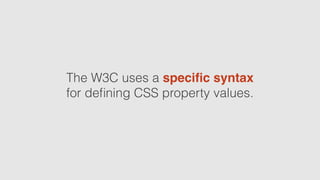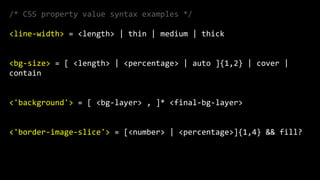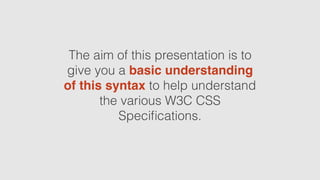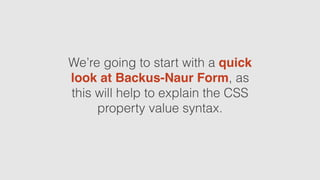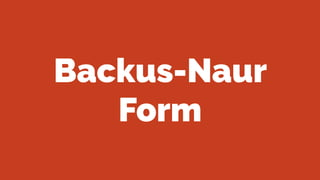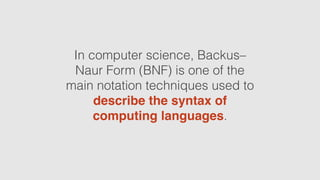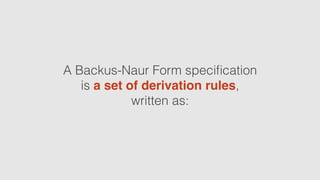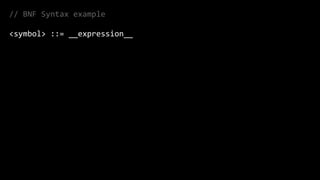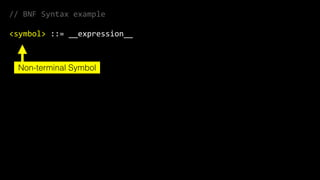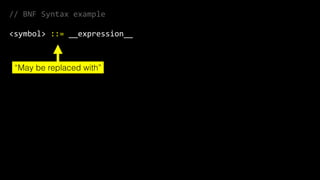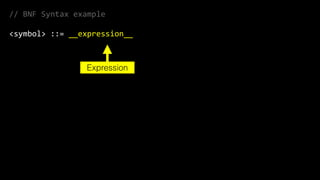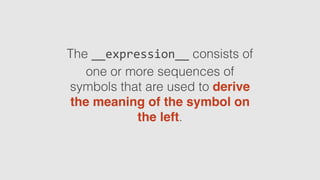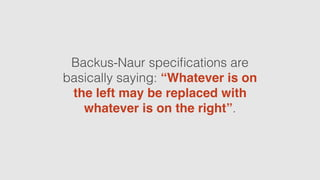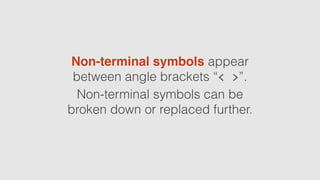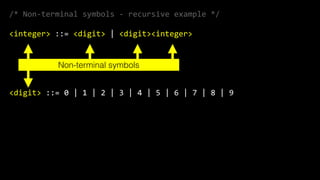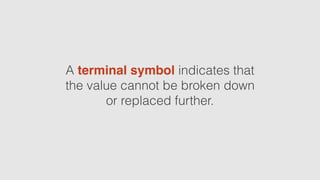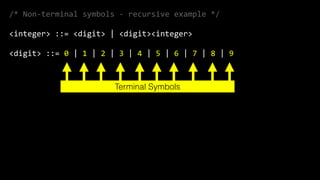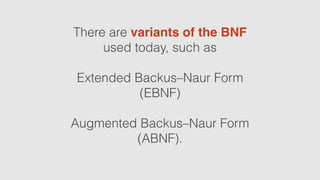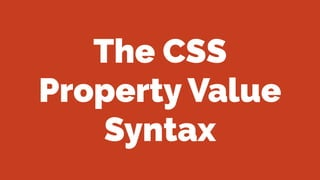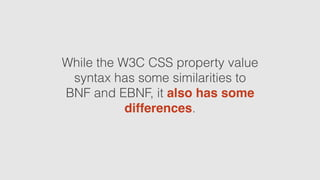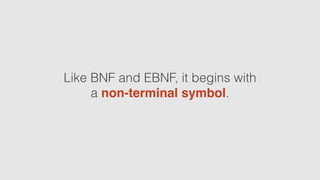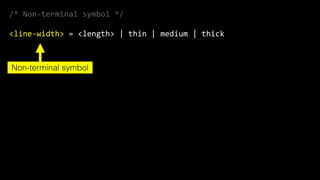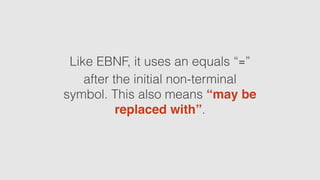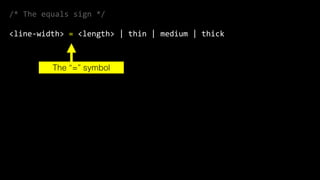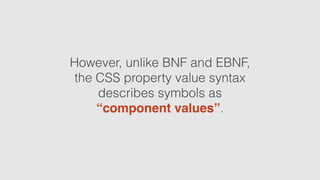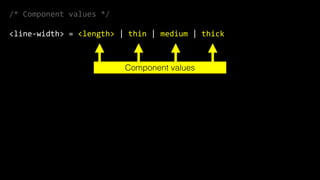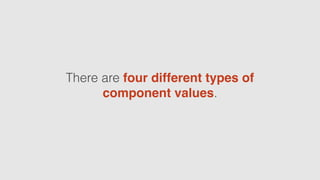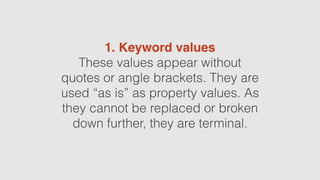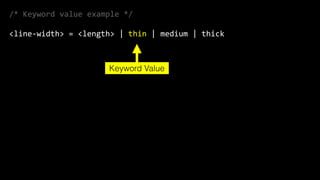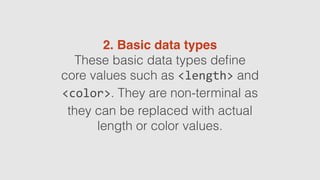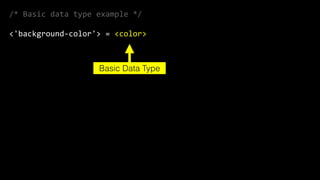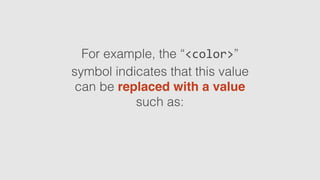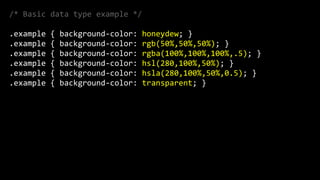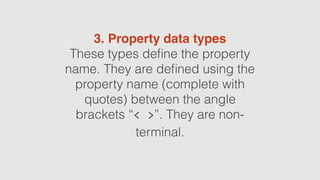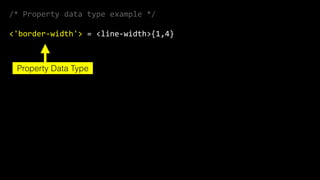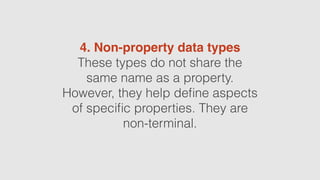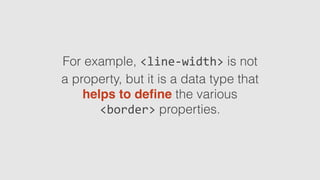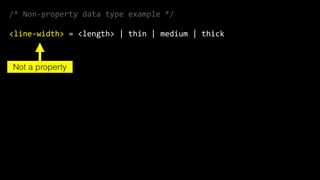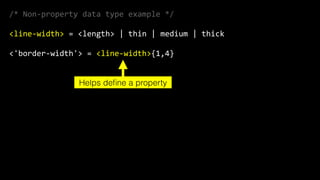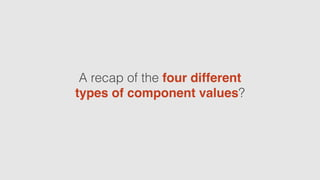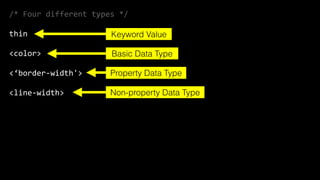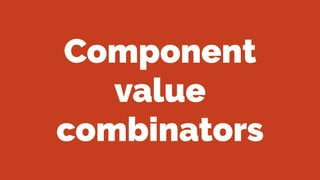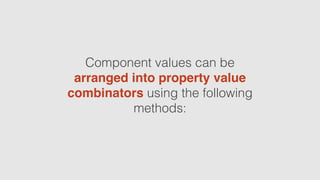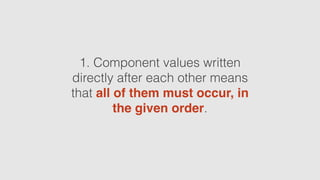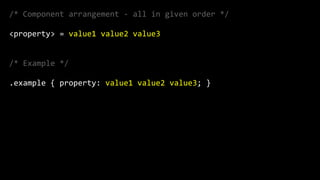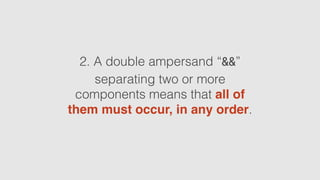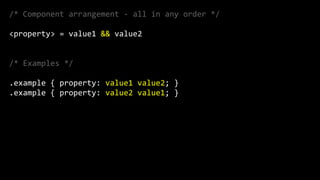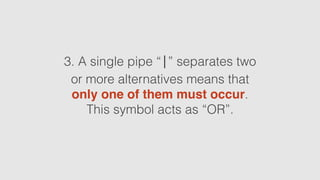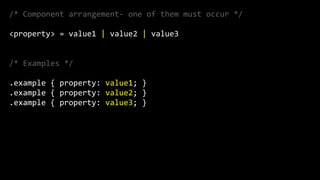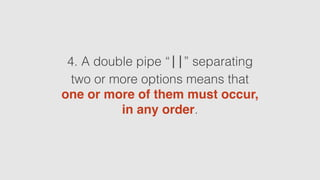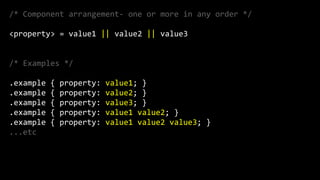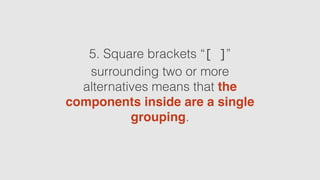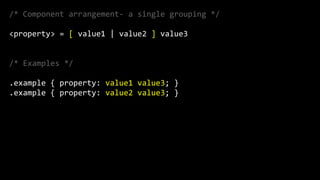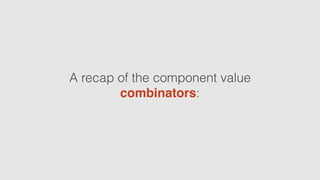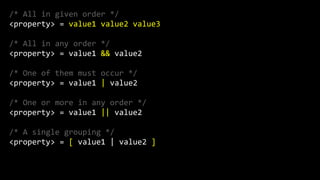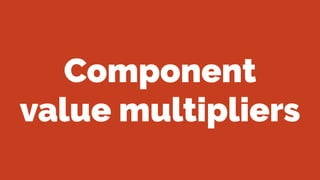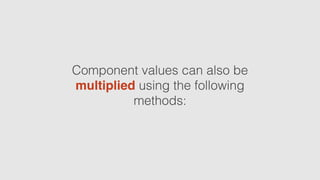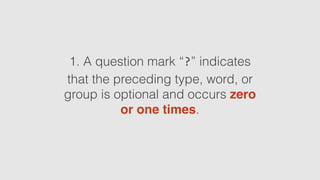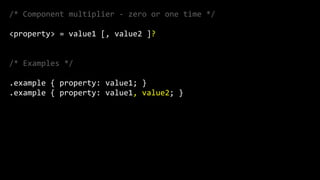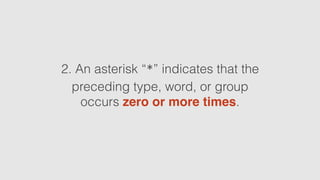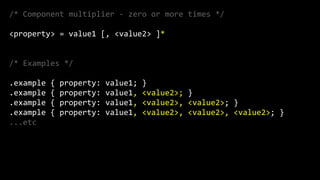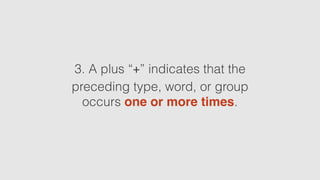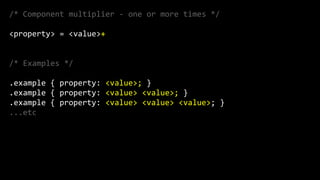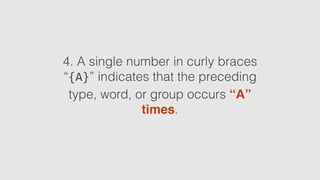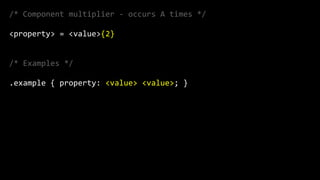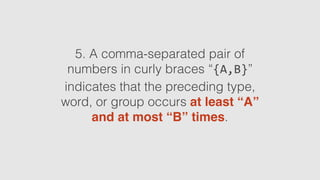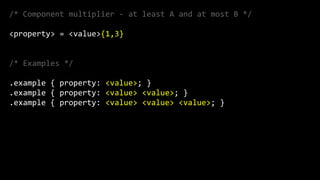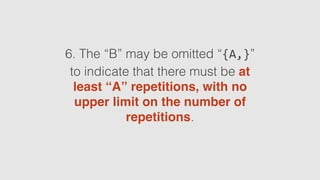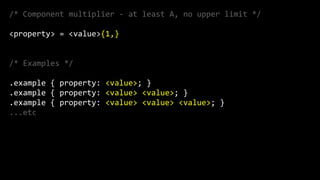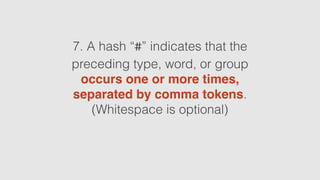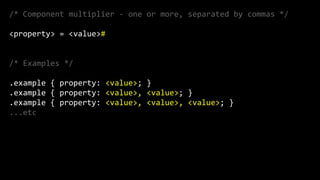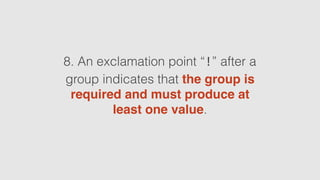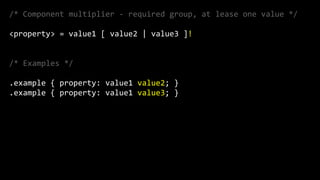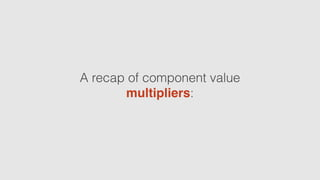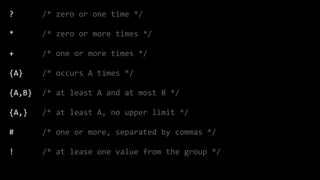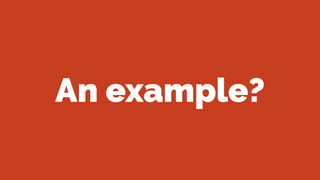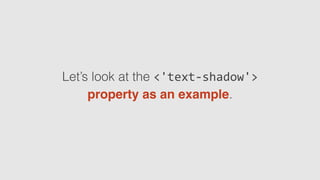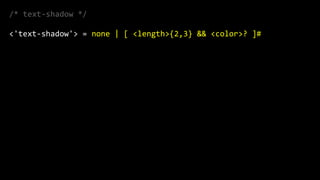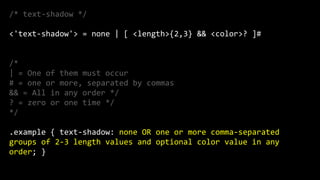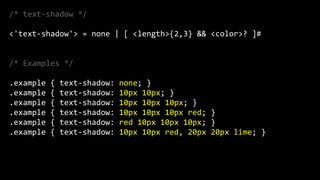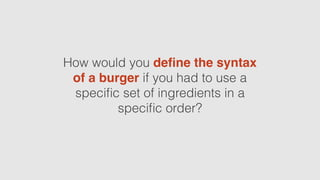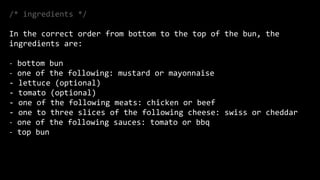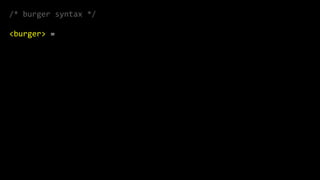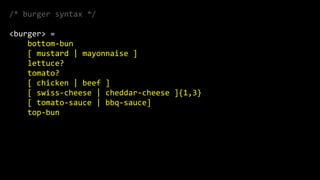1 sur 90
Publicité

### Understanding the mysteries of the CSS property value syntax

1. syntax. Understanding the mysteries of the CSS property value
2. The W3C uses a speciﬁc syntax for deﬁning CSS property values.
3. /*  CSS  property  value  syntax  examples  */   <line-­‐width>  =  <length>  |  thin  |  medium  |  thick   <bg-­‐size>  =  [  <length>  |  <percentage>  |  auto  ]{1,2}  |  cover  |   contain   <'background'>  =  [  <bg-­‐layer>  ,  ]*  <final-­‐bg-­‐layer>   <'border-­‐image-­‐slice'>  =  [<number>  |  <percentage>]{1,4}  &&  fill?
4. The aim of this presentation is to give you a basic understanding of this syntax to help understand the various W3C CSS Speciﬁcations.
5. We’re going to start with a quick look at Backus-Naur Form, as this will help to explain the CSS property value syntax.
6. Backus-Naur Form
7. In computer science, Backus– Naur Form (BNF) is one of the main notation techniques used to describe the syntax of computing languages.
8. A Backus-Naur Form speciﬁcation is a set of derivation rules, written as:
9. //  BNF  Syntax  example   <symbol>  ::=  __expression__
10. //  BNF  Syntax  example   <symbol>  ::=  __expression__ Non-terminal Symbol
11. //  BNF  Syntax  example   <symbol>  ::=  __expression__ “May be replaced with”
12. //  BNF  Syntax  example   <symbol>  ::=  __expression__ Expression
13. The __expression__ consists of one or more sequences of symbols that are used to derive the meaning of the symbol on the left.
14. Backus-Naur speciﬁcations are basically saying: “Whatever is on the left may be replaced with whatever is on the right”.
15. Non-terminal symbols appear between angle brackets “<  >”. Non-terminal symbols can be broken down or replaced further.
16. /*  Non-­‐terminal  symbols  -­‐  recursive  example  */   <integer>  ::=  <digit>  |  <digit><integer>   <digit>  ::=  0  |  1  |  2  |  3  |  4  |  5  |  6  |  7  |  8  |  9 Non-terminal symbols
17. A terminal symbol indicates that the value cannot be broken down or replaced further.
18. /*  Non-­‐terminal  symbols  -­‐  recursive  example  */   <integer>  ::=  <digit>  |  <digit><integer>   <digit>  ::=  0  |  1  |  2  |  3  |  4  |  5  |  6  |  7  |  8  |  9 Terminal Symbols
19. There are variants of the BNF used today, such as Extended Backus–Naur Form (EBNF) Augmented Backus–Naur Form (ABNF).
20. The CSS Property Value Syntax
21. While the W3C CSS property value syntax has some similarities to BNF and EBNF, it also has some differences.
22. Like BNF and EBNF, it begins with a non-terminal symbol.
23. /*  Non-­‐terminal  symbol  */   <line-­‐width>  =  <length>  |  thin  |  medium  |  thick Non-terminal symbol
24. Like EBNF, it uses an equals “=” after the initial non-terminal symbol. This also means “may be replaced with”.
25. /*  The  equals  sign  */   <line-­‐width>  =  <length>  |  thin  |  medium  |  thick The “=” symbol
26. However, unlike BNF and EBNF, the CSS property value syntax describes symbols as “component values”.
27. /*  Component  values  */   <line-­‐width>  =  <length>  |  thin  |  medium  |  thick Component values
28. There are four different types of component values.
29. 1. Keyword values These values appear without quotes or angle brackets. They are used “as is” as property values. As they cannot be replaced or broken down further, they are terminal.
30. /*  Keyword  value  example  */   <line-­‐width>  =  <length>  |  thin  |  medium  |  thick Keyword Value
31. 2. Basic data types These basic data types deﬁne core values such as <length> and <color>. They are non-terminal as they can be replaced with actual length or color values.
32. /*  Basic  data  type  example  */   <'background-­‐color'>  =  <color> Basic Data Type
33. For example, the “<color>” symbol indicates that this value can be replaced with a value such as:
34. /*  Basic  data  type  example  */   .example  {  background-­‐color:  honeydew;  }   .example  {  background-­‐color:  rgb(50%,50%,50%);  }   .example  {  background-­‐color:  rgba(100%,100%,100%,.5);  }   .example  {  background-­‐color:  hsl(280,100%,50%);  }   .example  {  background-­‐color:  hsla(280,100%,50%,0.5);  }   .example  {  background-­‐color:  transparent;  }
35. 3. Property data types These types deﬁne the property name. They are deﬁned using the property name (complete with quotes) between the angle brackets “<  >”. They are non- terminal.
36. /*  Property  data  type  example  */   <'border-­‐width'>  =  <line-­‐width>{1,4} Property Data Type
37. 4. Non-property data types These types do not share the same name as a property. However, they help deﬁne aspects of speciﬁc properties. They are non-terminal.
38. For example, <line-­‐width> is not a property, but it is a data type that helps to deﬁne the various <border> properties.
39. /*  Non-­‐property  data  type  example  */   <line-­‐width>  =  <length>  |  thin  |  medium  |  thick Not a property
40. /*  Non-­‐property  data  type  example  */   <line-­‐width>  =  <length>  |  thin  |  medium  |  thick     <'border-­‐width'>  =  <line-­‐width>{1,4} Helps deﬁne a property
41. A recap of the four different types of component values?
42. /*  Four  different  types  */   thin   <color>   <‘border-­‐width'>   <line-­‐width> Keyword Value Basic Data Type Property Data Type Non-property Data Type
43. Component value combinators
44. Component values can be arranged into property value combinators using the following methods:
45. 1. Component values written directly after each other means that all of them must occur, in the given order.
46. /*  Component  arrangement  -­‐  all  in  given  order  */   <property>  =  value1  value2  value3   /*  Example  */   .example  {  property:  value1  value2  value3;  }
47. 2. A double ampersand “&&” separating two or more components means that all of them must occur, in any order.
48. /*  Component  arrangement  -­‐  all  in  any  order  */   <property>  =  value1  &&  value2   /*  Examples  */   .example  {  property:  value1  value2;  }   .example  {  property:  value2  value1;  }
49. 3. A single pipe “|” separates two or more alternatives means that only one of them must occur. This symbol acts as “OR”.
50. /*  Component  arrangement-­‐  one  of  them  must  occur  */   <property>  =  value1  |  value2  |  value3   /*  Examples  */   .example  {  property:  value1;  }   .example  {  property:  value2;  }   .example  {  property:  value3;  }
51. 4. A double pipe “||” separating two or more options means that one or more of them must occur, in any order.
52. /*  Component  arrangement-­‐  one  or  more  in  any  order  */   <property>  =  value1  ||  value2  ||  value3   /*  Examples  */   .example  {  property:  value1;  }   .example  {  property:  value2;  }   .example  {  property:  value3;  }   .example  {  property:  value1  value2;  }   .example  {  property:  value1  value2  value3;  }   ...etc
53. 5. Square brackets “[  ]” surrounding two or more alternatives means that the components inside are a single grouping.
54. /*  Component  arrangement-­‐  a  single  grouping  */   <property>  =  [  value1  |  value2  ]  value3   /*  Examples  */   .example  {  property:  value1  value3;  }   .example  {  property:  value2  value3;  }
55. A recap of the component value combinators:
56. /*  All  in  given  order  */   <property>  =  value1  value2  value3   /*  All  in  any  order  */   <property>  =  value1  &&  value2   /*  One  of  them  must  occur  */   <property>  =  value1  |  value2   /*  One  or  more  in  any  order  */   <property>  =  value1  ||  value2   /*  A  single  grouping  */   <property>  =  [  value1  |  value2  ]
57. Component value multipliers
58. Component values can also be multiplied using the following methods:
59. 1. A question mark “?” indicates that the preceding type, word, or group is optional and occurs zero or one times.
60. /*  Component  multiplier  -­‐  zero  or  one  time  */   <property>  =  value1  [,  value2  ]?   /*  Examples  */   .example  {  property:  value1;  }   .example  {  property:  value1,  value2;  }
61. 2. An asterisk “*” indicates that the preceding type, word, or group occurs zero or more times.
62. /*  Component  multiplier  -­‐  zero  or  more  times  */   <property>  =  value1  [,  <value2>  ]*   /*  Examples  */   .example  {  property:  value1;  }   .example  {  property:  value1,  <value2>;  }   .example  {  property:  value1,  <value2>,  <value2>;  }   .example  {  property:  value1,  <value2>,  <value2>,  <value2>;  }   ...etc
63. 3. A plus “+” indicates that the preceding type, word, or group occurs one or more times.
64. /*  Component  multiplier  -­‐  one  or  more  times  */   <property>  =  <value>+   /*  Examples  */   .example  {  property:  <value>;  }   .example  {  property:  <value>  <value>;  }   .example  {  property:  <value>  <value>  <value>;  }   ...etc
65. 4. A single number in curly braces “{A}” indicates that the preceding type, word, or group occurs “A” times.
66. /*  Component  multiplier  -­‐  occurs  A  times  */   <property>  =  <value>{2}   /*  Examples  */   .example  {  property:  <value>  <value>;  }
67. 5. A comma-separated pair of numbers in curly braces “{A,B}” indicates that the preceding type, word, or group occurs at least “A” and at most “B” times.
68. /*  Component  multiplier  -­‐  at  least  A  and  at  most  B  */   <property>  =  <value>{1,3}   /*  Examples  */   .example  {  property:  <value>;  }   .example  {  property:  <value>  <value>;  }   .example  {  property:  <value>  <value>  <value>;  }
69. 6. The “B” may be omitted “{A,}” to indicate that there must be at least “A” repetitions, with no upper limit on the number of repetitions.
70. /*  Component  multiplier  -­‐  at  least  A,  no  upper  limit  */   <property>  =  <value>{1,}   /*  Examples  */   .example  {  property:  <value>;  }   .example  {  property:  <value>  <value>;  }   .example  {  property:  <value>  <value>  <value>;  }   ...etc
71. 7. A hash “#” indicates that the preceding type, word, or group occurs one or more times, separated by comma tokens. (Whitespace is optional)
72. /*  Component  multiplier  -­‐  one  or  more,  separated  by  commas  */   <property>  =  <value>#   /*  Examples  */   .example  {  property:  <value>;  }   .example  {  property:  <value>,  <value>;  }   .example  {  property:  <value>,  <value>,  <value>;  }   ...etc
73. 8. An exclamation point “!” after a group indicates that the group is required and must produce at least one value.
74. /*  Component  multiplier  -­‐  required  group,  at  lease  one  value  */   <property>  =  value1  [  value2  |  value3  ]!   /*  Examples  */   .example  {  property:  value1  value2;  }   .example  {  property:  value1  value3;  }
75. A recap of component value multipliers:
76. ?   *   +   {A}   {A,B}   {A,}   #   ! /*  zero  or  one  time  */   /*  zero  or  more  times  */   /*  one  or  more  times  */   /*  occurs  A  times  */   /*  at  least  A  and  at  most  B  */   /*  at  least  A,  no  upper  limit  */   /*  one  or  more,  separated  by  commas  */   /*  at  lease  one  value  from  the  group  */
77. An example?
78. Let’s look at the <'text-­‐shadow'> property as an example.
79. /*  text-­‐shadow  */   <'text-­‐shadow'>  =  none  |  [  <length>{2,3}  &&  <color>?  ]#
80. /*  text-­‐shadow  */   <'text-­‐shadow'>  =  none  |  [  <length>{2,3}  &&  <color>?  ]#   /*     |  =  One  of  them  must  occur   #  =  one  or  more,  separated  by  commas   &&  =  All  in  any  order  */   ?  =  zero  or  one  time  */   */   .example  {  text-­‐shadow:  none  OR  one  or  more  comma-­‐separated   groups  of  2-­‐3  length  values  and  optional  color  value  in  any   order;  }
81. /*  text-­‐shadow  */   <'text-­‐shadow'>  =  none  |  [  <length>{2,3}  &&  <color>?  ]#   /*  Examples  */   .example  {  text-­‐shadow:  none;  }   .example  {  text-­‐shadow:  10px  10px;  }   .example  {  text-­‐shadow:  10px  10px  10px;  }   .example  {  text-­‐shadow:  10px  10px  10px  red;  }   .example  {  text-­‐shadow:  red  10px  10px  10px;  }   .example  {  text-­‐shadow:  10px  10px  red,  20px  20px  lime;  }
82. A burger challenge
83. (Disclaimer: This idea is ripped off from a great A List Apart article by J. David Eisenberg.) http://alistapart.com/article/readspec
84. How would you deﬁne the syntax of a burger if you had to use a speciﬁc set of ingredients in a speciﬁc order?
85. Anyone want to volunteer? The ﬁrst person to get the correct answer during the break will win a copy of “Offscreen” magazine. http://www.offscreenmag.com/
86. /*  ingredients  */   In  the  correct  order  from  bottom  to  the  top  of  the  bun,  the   ingredients  are:   -­‐ bottom  bun   -­‐ one  of  the  following:  mustard  or  mayonnaise   -­‐  lettuce  (optional)   -­‐  tomato  (optional)   -­‐  one  of  the  following  meats:  chicken  or  beef   -­‐  one  to  three  slices  of  the  following  cheese:  swiss  or  cheddar   -­‐ one  of  the  following  sauces:  tomato  or  bbq   -­‐ top  bun
87. /*  burger  syntax  */   <burger>  =
88. The answer is on the following screen.
89. /*  burger  syntax  */   <burger>  =          bottom-­‐bun          [  mustard  |  mayonnaise  ]          lettuce?          tomato?          [  chicken  |  beef  ]          [  swiss-­‐cheese  |  cheddar-­‐cheese  ]{1,3}          [  tomato-­‐sauce  |  bbq-­‐sauce]          top-­‐bun